Syllogism Shortcuts

Today I am going to share a systematic method to solve Syllogism questions. In every competitive exam, you will see at least 4-5 questions from this chapter.

I will start with explaining basic syllogism terminology which will be followed by practical examples.

AllSomeNOCase #   All - SomeLet!
You have 1000 \$ in your pocket.One of your friend needs 600 \$ to pay his Bill.He wants to borrow money from you.He comes to you and say,"Do you have 600 \$ ?.What would you say,"Afcoss Yes!".Even if He ask for 1 \$, or 999 \$ .Your answer will always "Yes!".
So Overall you had 1000 \$ which is called "All " or All of the money you had & What is your friend want some of the money like here 600 or it could be 1\$ or 999 \$ and what we call that some portion of money out of it.or " Some".

That's why In " All " Case, "Some" is always true.

Example:

Statement : All A are B.
Possible Diagram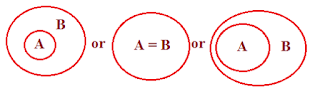Conclusion
All A are B
Some A are B
Some B are A
Some A are not B
Some B are not A   ✘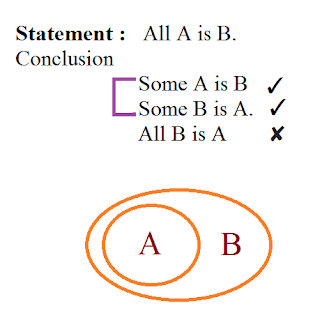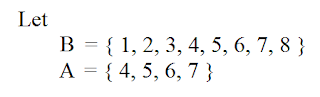Now Here we can see that A contains set of numbers. So we call it is as Set A and Same for B 'Set B'.
All the numbers in A is contained by B hence All A is B true But All the numbers in B is not in A or not contain by A so All B is A → False.

Now Let understand How All A is B → True But All B is A  False
Let  Box A & Box B . Here We can see that Box B is inside the Box A Hence Box B can fit into Box A but Box A cannot fit into Box B.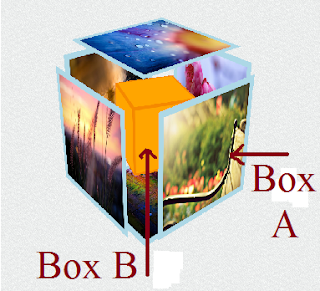Case #    Some - Some NotAssume you have given the exam & you passed with some marks that mean you got some marks and some or not. Even if you passed the exam with 99% marks still you missed the 1%. So Even 99% is count under the same case.and 1% is also counted in some case.hence In 'Some' case 'Some Not' is true.

Statement : Some A are B
Possible DiagramConclusion

All B are A
Some B are A
No A is B

Statement : Some A are not B
Possible Diagram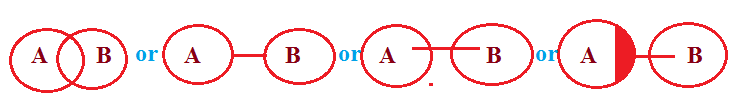ExplanationHere we see that Set A & Set B has some common values hence we can say that Some A is B & Some B is A But there also some values which are not common in both A & B.So We can also say that Some A are not B & Some B are not A.

Case #    No- Some Not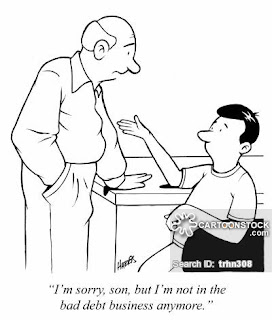Assume You have no money in your pocket.So if you got no money.It means also that some money is not in your pocket.Having No means also some not.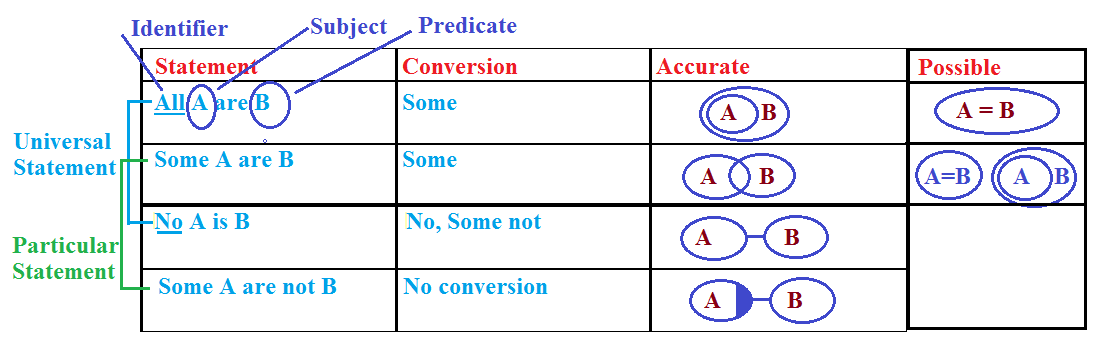Point to Remember while Solving Syllogism

• Anything is possible in a statement.                                                                                                        like  Some pens are fans. No fan is heater
• Negative Answer will never be there.

Simple Case

 Statement Conclusion Some All , No ,  [ False ] Some,Some Not [ True ] All No , Some Not [ False ] Some , All [ True ] Some Not Only Statement [ True ] No Some , All [ False ] No , Some Not [ True ]

Possibility
 Statement Conclusion Some No , Some [ False ] All , Some Not [ True ] All ✘ No ✘ Some Not Some Not , All [ False ] Some , No [ True ]

Some Examples

Example 1

Statement : Some A are B, Some B are C , All C are DConclusion
Some A are not D ✘
Some B are D
Some A are C       ✘

Example 2

Statement: Some A are B, Some B are C, No C is DConclusion
Some B are not D  ✘
Some D are not B  ✘
Some A are C        ✘

Example 3

Statement: All A are B , All A are C , All A are D , No D is E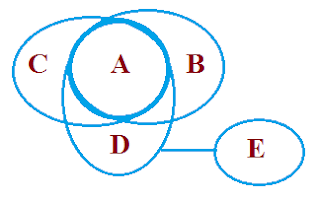Conclusion:
Some B are not E
Some A are not E
No C is E
No A is E
No E is A

Example 4

Statement: Some A are B, Some B are C, Some C are D, Some D are E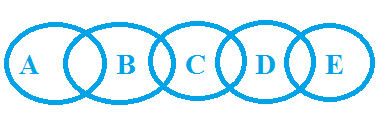Conclusion :
Some C are not A  ✘
Some B are not D  ✘
Some A are E        ✘
All B are D            ✘
No A is E               ✘
Some C are A        ✘
All B are E            ✘
No B is E              ✘

' Either Or ' & ' Neither Nor '

Complementary Pair

1. If One Conclusion is positive & One is Negative

No - Some
Some - Some Not
All - Some Not

2.  Same Subject & Same Predicate are there
3.  & not able to draw both the conclusion then answer will be Either Or

Example

Example 1

Statement : All A are B , All A are C , All A are D , No D is E, Some F is C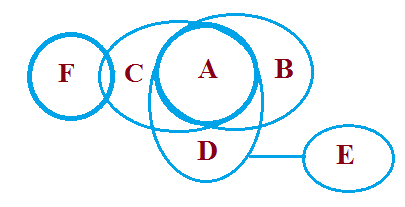Conclusion :-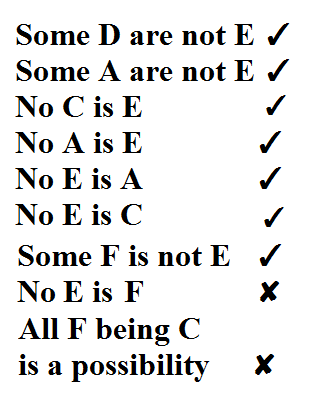Example 2

Statement : Some A are B, Some B are C, Some C are D, Some D are EConclusion :Exception
1. In case of Universal (like 'All & No' ) Conclusion Complementary pair does not work
2. In this type of Question " Neither Nor "

Example 3

Statement: Some A are B, All B are C, No C is DConclusion :
Some A are not D    ✘
No B is D                ✘
Some A are C           ✘
Some D are B           ✘

Example 4

Statement: All A are B, No B is C , All C are D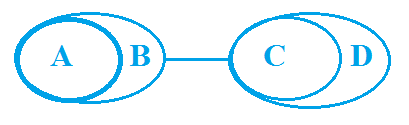Conclusion:
Some A are not D ✘
No D is B             ✘
No A is C
No A is D
No D is A            ✘

PossibilityStatement Some A are B, Some B are C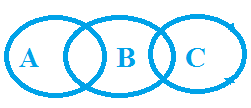Conclusion

Explanation (Simple case A,B & C) :

Let A, B & C are three person. A & B know each other. B & C know each other But B knows both.Now, Will A talk about C? Answer is 'Not!,Neither Positive Nor Negative' because he doesn't know him.So conclusion C is False.

Possibility Case (Conclusion : D,E & F) :

Now Let's take a look at conclusion E.Now Suppose there is raining outside your home and someone comes to you and say there is a possibility to rain today.What would you say to him,'Mad!"The Same scenario is here By looking at diagram it clearly says that Some B are A then how could say it is 'possible' which is actually there.Hence Possibility makes it wrong or false the conclusion.So Conclusion E is wrong.

Now talk about conclusion F which says Some A are not c which we don't know but it says to let it is a 'possibility' so anything could be possible which doesn't make our statement wrong or contradict with statement.If we make it like this below:We talk here about possibility which could be anything which especially not contradict with our statement.So F which was False in Simple Case but When we talk about the possibility is becoming true.

Let's take another example for this.Now Let There is a cloudy sky outside your home.Someone comes to you and say," There is a possibility that there might be rain today", Now you have no idea whether it rain today or not.So you might be saying ," Bro! It is possible".Now here you cannot talk about negative because in Syllogism Negative Answer will never be there.

In a statement word like these called possibilities
• Can be
• Possible
• May be / might be
• Chances
• Occurs
• is being a possibility
• is a possible
In a statement word like these called sureties
• Can Never be
• Can

Find Other Articles on SyllogismWhat's trending in BankExamsToday

Smart Prep Kit for Banking Exams by Ramandeep Singh - Download here1.In third diagram ,can we say all b are a?

2.thank u sir

3.sir please provide some tricks for boat stream, train and mensuration

4.These topics are lined up for next week

5.In this Diagram Yes!
It is true that All B are A

6.But given false

7.But given false.

8.These are possible diagrams for some A are B.In all these diagrams Some is true so we can choose any one diagram while solving possibility.It is false Because in statement like some A is B all the conclusion will wrong like ( All, No)except like ' some & some not ' while solving simple case.

9.no.. some A are B doesnt imply all B are A

Some A are B simply implies Some B are A.. so d answer gyvn as false is correct

10.Thank you so much! super tricks! please provide more exercises! easy as well as critical stage! relay helpful!

11.12.In this diagram we can say that
All A is B [ True ]
Some A is B [ True ]
Some B is A [ True ]
So If statement is this ' Some A is B '
we can also make this diagram as well because it cannot contradict our statement

13.Thnx sir
Sir plz provide short tricks for machine input also

14.ma'm.. i am sorry to inform u but thn der are some venn diagrams one needs to consider while solving these syllogisms.. i have uploaded d diagrams wid dis comment..

When in a given premise , only the variables are interchanged , its d case of implication and to solve such qns considering the feasibility and applicability all the venn diagrams i.e our answer must be universally correct..

In the premise : Some A are B , if we consider all its venn diagrams , then Some B are A is the only possiblity which fits for all cases , rest others donot..

Actually , my explanation may be rudimentary coz der r some sets of steps I follow , which is too long to write and explain here.. but thn if u can cross-check it from any source which is widout errors, then u may do so..

15.Thanks a lot 😊 ,sir.

16.Solanki DharmeshJune 13, 2015 at 5:26 PM

sir you joying all the student in whatsUP,..THAT'S GOOD HELPING FOR ALL STUDENT ...

17.Thank u Raman sir

18.Good Question.
Like you say Some B are A is only possible .Yup if we follow through all it's diagram but we don't need to do that.we need to follow only Accurate Daigram which is this for some case as in picture . The other possible diagram will help us in question like possibility where we need to find whether the given possibility will became possible without contradicting our statement.Lets take an example Where Statement says All A are B now Here Some B is A can also be true but As you say
If statement goes like like this All B are A
then Conclusion Some B or A cannot be possible so it help us to find whether the possible can became true without changing or modifying the statement or question.

But You are still right about it that this diagram make us confuse in this particular case . So i edited now.

19.yes.. exactly.. der r 2 cases - conversion and implication..

conversion is when d variables are variables are interchanged and implication is when variables are not interchanged..

acc 2 d snap dat u hav uploaded , it says dat Some A are B means Some B are A.. i.e All B are A will be wrong but All A being B is a possiblity is correct

20.If there Is diagram of All B are A then
these following conclusion will true
Conclusion:
Some A is B [True]
Some B is A [ True ]
All A is B [ True ]
But
All B is A [ False]
In All case Some will True

21.Good work we need this type info.. Keep doing

22.ma'm.. acc to u , if All B are A is given , then All B is A will be false ?? how ??

also., if All B are A , then All A are B is false, it shud be Some A are B.. venn diagram says it..

23.okk okk... thanku ma'm.. plzz do com up wid tricks from machine input and output.. and i am sorry if i became rude to u.. sorry

24.Priya Mam, I've confusion in conclusion D n E in possibility case.

25.Dear Sir/ Madam, If statement and conclusion both are same, then what will be the answer? is it true or false.

26.bt how dat will be true...............All B are A. Statement never be conclusion ...............please explain it

27.thank u sir..really a superb explanation..sir pdf ke questions chahiye abb

28.WoWWW!!! super explanation.. for the past two weeks i have been searching about this clear explantion. Now i got it!!! Thank u Raman Sir

29.All A are B , All A are C , All A are D , No D is E.

in this case No C is E will be true na... u havementioned false.. plz explain :)

30.Example 3
Statement: All A are B , All A are C , All A are D , No D is E
syllogism example tricks
Conclusion:
Some B are not E ✓
Some A are not E ✓
No C is E ✓
No A is E ✓
No E is A ✓ can somone tell me how is the first conclusion wrong. i shouldn't have come here, i got soo confused

31.If All A are B.
Then How Some b are not A is false?

Accn to Venn diagram, it holds true.

Thanks for commenting. Follow us on Telegram. Search BankExamsToday